Chemguide: Support for CIE A level Chemistry ``` ``` Paper 5 Formulae and equations Writing formulae Writing formulae is one of the basic skills in chemistry, and it is disturbing when an Examiner's Report says that many candidates had problems with the formulae of simple compounds. One question (June 2014 Papers 51 and 53 Q1) told you that when magnesium nitrate(V) is heated, it decomposes to form magnesium oxide, nitrogen(IV) oxide and oxygen, and asked you to write an equation for the reaction. Apparently many candidates couldn't do this. I wonder whether the problem here is just in the use of the oxidation states (V) and (IV). If, instead of these, you had just been asked about magnesium nitrate and nitrogen dioxide, could you have written the correct formulae for the two and then produced the right equation for the reaction? If not, then you have got a serious problem which you must get sorted out. Let's deal first with the oxidation states in the names. There are two familiar anions containing nitrogen and oxygen: NO3-, commonly called the nitrate ion NO2-, commonly called the nitrite ion There is no simple way of working these out - you just have to learn them. The modern names of these both use the word "nitrate", and then include the oxidation state of the nitrogen. So . . . NO3- is now the nitrate(V) ion NO2- is now the nitrate(III) ion But, in truth, most people probably still use the old names. And knowing the oxidation states doesn't help you with the formulae, because these names don't tell you anything about the number of oxygens or the charge on the ion. So you have to learn these names and formulae. Note:  Actually, if you were given the full modern name, which also counts the oxygens, you could work out the charge on the ion. What we normally call the nitrate ion is the trioxonitrate(V) ion. If you could be bothered, you could work out that such an ion has a single negative charge from the oxidation states. The nitrogen(IV) oxide case is less of a problem because this is an uncharged compound, not an ion. The oxidation state of oxygen is nearly always -2. And if the compound is uncharged, the sum of the oxidation states has to be zero. There must therefore be 2 oxygens (2 x -2) to balance the nitrogen (+4): NO2, commonly called nitrogen dioxide. Note:  How do you know that the nitrogen is +4 and not -4? Because the most electronegative element in a compound always has the negative oxidation state, and oxygen is more electronegative than nitrogen. The other common cases which are similar are: SO42-, the sulfate ion or now the sulfate(VI) ion SO32-, the sulfite ion or now the sulfate(IV) ion SO2, sulfur dioxide or now sulfur(IV) oxide SO3, sulfur trioxide or now sulfur(VI) oxide Is this all a complete pain in the neck with these common substances? Yes! Just learn the alternatives. ``` ``` So . . . can you write the formula for magnesium nitrate(V) without really thinking about it? (Answer at the bottom of the page.) If you got it wrong, or even had to struggle to get the right answer, then you need urgently to practise writing formulae. This is all covered in detail in the first chapter of my calculations book. If you don't have access to a copy, some of this chapter together with its answers is currently available from Amazon's "Look inside" feature. Click on the book image at the top left of the page that this link will take you to. The amount is fairly limited. ``` ``` Writing equations Full equations for simple reactions This involves just writing down the formulae for everything and then balancing the equation. This is also in the first chapter of my book, and is currently available in the "Look inside" feature - see above. If you have problems, then practise. Don't forget that you may have to include state symbols - be guided by what the examiners are doing or saying. Ionic equations You should be prepared to write ionic equations for simple reactions involving, for example, precipitation or acid-base reactions. Precipitation reactions happen when you mix two solutions containing ions and get a solid formed. You start the equation from the right-hand side. All you need to do is write down the formula of the precipitate on the right-hand side of the equation, and add the state symbol (s). Then do the left-hand side of the equation. Write down the formulae of the ions which came together to produce that precipitate. This time the state symbols will be (aq) because these reactions always involve ions in solution. For example, in the formation of a precipitate of barium sulfate by mixing solutions containing a soluble barium salt and a soluble sulfate: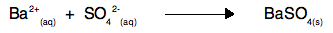Be careful to balance the equation where necessary. The next equation comes from a past CIE paper involving the precipitation of nickel(II) iodate. You were given the formula for the iodate ion and asked to write the ionic equation.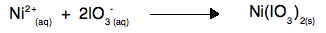Note:  There was a nasty sting in this question, because the source of the iodate ions was solid potassium iodate. However, if you gave the state symbol (s) for the iodate ions, you lost the mark for the question. You had to realise that precipitation reactions involve solutions of ions, and that the potassium iodate first dissolved in the water, and then the iodate ions reacted with nickel(II) ions. It is fairly obvious if someone points it out to you, but easy to miss in an exam. For acid-base reactions, there are a few common ionic equations that you should be familiar with, and you might need to work out other ones.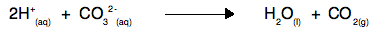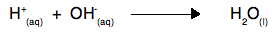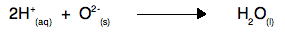You must be careful with the state symbols for the carbonate, hydroxide or oxide ions - they might be solid or in solution. Read the question carefully. What these equations say is that any acid will react with anything containing carbonate, hydroxide or oxide ions to give the products shown. ``` ``` Writing ionic equations for redox reactions by first working out electron-half-equations for the oxidation process and the reduction process separately is an essential skill in A level chemistry and is covered on the Chemguide page Writing ionic equations for redox reactions. That page mainly covers writing equations for these reactions in acidic conditions. There is another page describing how to do it in alkaline conditions. Don't even think about reading that second page unless you are totally confident about what you read on the first page. When you are sure that you understand the first page, I want to talk about a Paper 5 question which the Examiner's Report said few candidates could do. That is ridiculous - this is a key, and not very difficult, skill that all A level chemistry students should have. The question was June 2015 papers 51 and 53 Q1. You were asked to write half-equations for the changes from methanoate ions, HCOO-, being oxidised to CO2 by MnO4- which was reduced to Mn2+ in the process. You were told that the reaction was done under acid conditions. All this information was given to you in the question. Working out the half-equation for the reaction from MnO4- to Mn2+ under acid conditions was described in detail on the page you should just have read and understood. I am not repeating it now, but you should work it out for yourself without referring back to that page until you are sure you have a right answer. Now we will work out the half-equation for the methanoate ions being oxidised to carbon dioxide. Start by writing down what you know. You are told that methanoate ions end up as carbon dioxide.Now check the balancing of everything apart from oxygens and hydrogens. In this case, that only leaves the carbon, and that is OK. All you are allowed to add to equations in acid conditions is water (if you need to balance oxygens) and hydrogen ions (if you need to balance hydrogens) and electrons. The oxygens are fine, so you just need to balance the hydrogens. Leave the problem of the charges until the final step. You can do that by adding a hydrogen ion to the right-hand side.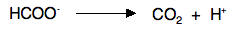Now balance the charges by adding electrons. There is at present a negative charge on the left-hand side, and a positive one on the right. Add some electrons on the right-hand side to make that a single negative charge overall.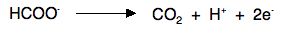So . . . is that difficult? Absolutely not! So why did the Examiner's Report say ". . . the correct methanoate equation was very rarely seen."? The Report also said "Most candidates who gained partial credit usually did so with the manganate(VII) half equation . . .". I find this very strange, and can only think of one explanation for it (but I could be wrong). The manganate(VII) half-equation comes up quite commonly in, for example, working out equations for titrations, and I wonder if students are simply being told to learn the manganate(VII) half-equation rather than understanding how to work it out. That is a seriously bad idea, especially with an Exam Board like CIE who are more likely to ask questions which test your understanding rather than random bits of learning. If you spend a bit of time understanding how it works, it will save you a lot of pointless learning which probably won't cover what you are going to be asked anyway! If you fall into this category, go back and spend enough time on the last link above to be sure that you could answer any similar question that might come up in the future because you understand how to work these equations out. It didn't come up in the question we have been looking at, but you must also understand how to add these equations together to come up with an overall ionic equation. That is also covered on the same page. ``` ``` The formula for magnesium nitrate(V):  Mg(NO3)2 ``` ``` Go to the Paper 5 Menu . . . To return to the Paper 5 menu Go to the CIE Main Menu . . . To return to the list of all the CIE sections Go to Chemguide Main Menu . . . This will take you to the main part of Chemguide. © Jim Clark 2017